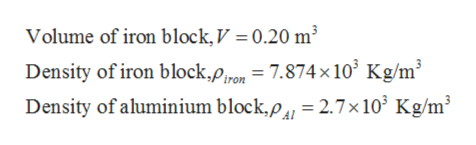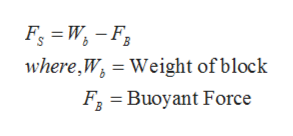# An iron block of volume 0.20 m3 is suspended from a spring scale and immersed in a flask of water. Then the iron block is removed, and an aluminum block of the same volume replaces it. (a) In which case is the buoyant force the greatest, for the iron block or the aluminum block? (b) In which case does the spring scale read the largest value? (c) Use the known densities of these materials to calculate the quantities requested in parts (a) and (b). Are your calculations consistent with your previous answers to parts (a) and (b)?

Question
54 views

An iron block of volume 0.20 m3 is suspended from a spring scale and immersed in a flask of water. Then the iron block is removed, and an aluminum block of the same volume replaces it. (a) In which case is the buoyant force the greatest, for the iron block or the aluminum block? (b) In which case does the spring scale read the largest value? (c) Use the known densities of these materials to calculate the quantities requested in parts (a) and (b). Are your calculations consistent with your previous answers to parts (a) and (b)?

check_circle

Step 1

Given,help_outlineImage TranscriptioncloseVolume of iron block, V = 0.20 m³ %3D Density of iron block,pron = 7.874×10° Kg/m³ Density of aluminium block,p, = 2.7×10³ Kg/m³ fullscreen
Step 2

Part (a):

Buoyant force will be acting on an object that is immersed in liquid and is equal to weight of fluid displaced. It is given that volume of aluminum and iron blocks are same so weight displaced will be equal for both blocks. Hence buoyant force will be equal for both blocks.

Step 3

Part (b):

Buoyant force will be acting upwards while weight will be acting downwa...help_outlineImage TranscriptioncloseFg = W, – F3 where,W, = Weight of block F, = Buoyant Force fullscreen

### Want to see the full answer?

See Solution

#### Want to see this answer and more?

Solutions are written by subject experts who are available 24/7. Questions are typically answered within 1 hour.*

See Solution
*Response times may vary by subject and question.
Tagged in

### Fluid Mechanics